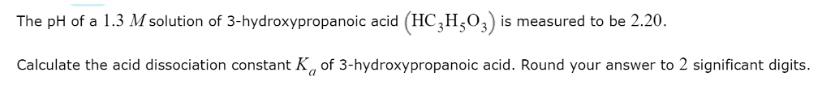# Problem: The pH of a 1.3 Absolution of 3-hydroxypropanoic acid is (HC3H5O3)measured to be 2.20. Calculate the acid dissociation constant Ka of 3-hydroxypropanoic acid. Round your answer to 2 significant digits.

###### FREE Expert Solution
79% (257 ratings)###### Problem Details

The pH of a 1.3 Absolution of 3-hydroxypropanoic acid is (HC3H5O3)measured to be 2.20.

Calculate the acid dissociation constant Ka of 3-hydroxypropanoic acid. Round your answer to 2 significant digits.